P2 Edge Roadsteer Does LOGO Language — Parallax Forums

# P2 Edge Roadsteer Does LOGO Language

LOGO is a simple language designed to teach basic programming with movement. Instead of using turtle graphics I am using my RoadSteer unit which has two NEMA 17 stepper motors to drive it. This seems to be a good fit for LOGO as I can calculate the exact distance the unit travels based on the number of steps.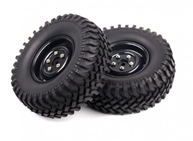First let’s do some calculations. We have two tires that are 50mm in size. Converting mm to inches we get 1.9685 inches. The stepper motors turn at 1.8 degrees per step or 200 steps per revolution. I have the A4988 stepper set to 16th step position drive so it will take 3200 step to do one revolution. So to calculate the distance traveled would be to find the circumference of the wheels and divide it into the number of steps to get steps per inch.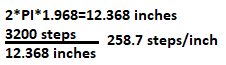So to move forward 10 inches we need to step about 2587 times. To keep things simple we will use 259 as are step count. In this video we will tell the robot to move forward 20 inches and see if it gets there. Then we will move back 20 inches and see if it returns to home.
Forward Movement
We can see from the video that the robot did not reach 20 inches and was about 1 inch short.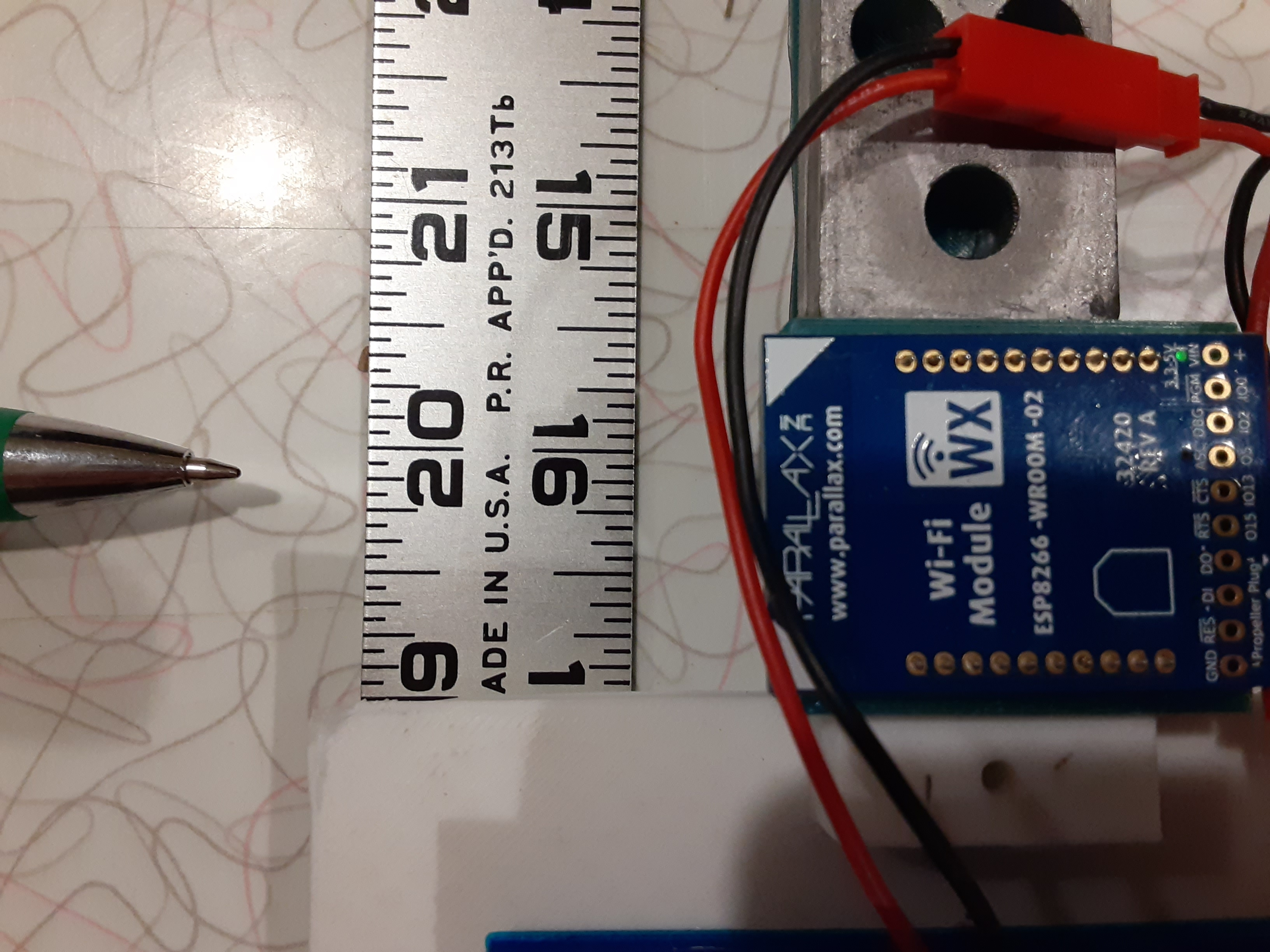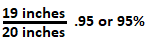So we need to add 5% to our step count to get it to 20 inches. 259 + 5% is about 272 rounding up. Let’s try this value and see what happens.
Forward Movement 2
Now we need to figure out how to turn. So if we need to turn 90 degrees I need to know how many steps to turn each wheel. Since we are going to spin the RoadSteer in place each wheel will move in the opposite direction. This makes it a circle which we can calculate just like before. The wheels are separated by about 6.75 inches from the center of threads.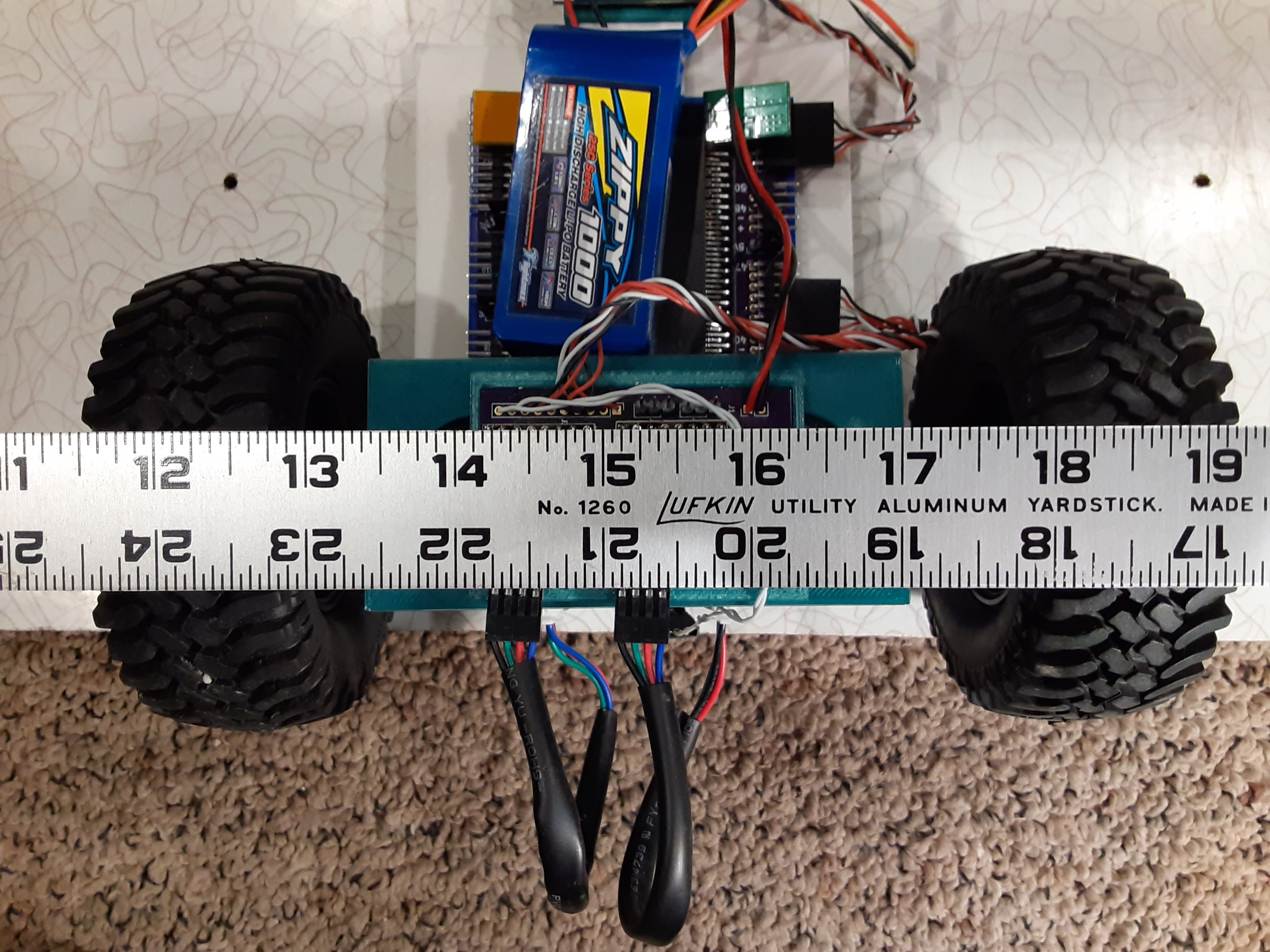Since we are spinning in place that would be the diameter of the circle so we will divide by 2.5726 steps is equal to 360 degrees so for each degree we will divide it by 360. To turn 90 degrees we would use.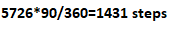To turn 90 degrees would be about 1431 steps. Since we need to know if actually gets to 90 degree we will instead use 360 degrees. If the Roadsteer turns completely around we will see it. Let’s take a look.
Circle 360
I can see by the pointer it did not reach 360 degrees but looks close. Adding about 10 degrees got it to 360 degrees.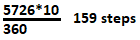So my new value is 5726 + 159 = 5885. This seem to work so now my basic LOGO functions are working.
Need to finish up the TO function and the save and load functions.

```#include <stdbool.h>
#include <stdio.h>
#include <string.h>
#include <propeller.h>
#include "json.h"
#include "step.h"

void DoLogo(void);
void DoParse(void);
int FindW(char *);
int FindT(char *);
void DoForward(void);
void DoBack(void);
void DoLeft(void);
void DoRight(void);
void DoRepeat(void);
void DoBye(void);
void DoSave(void);
void DoTo(void);
void DoSleep(void);
void DoRunTo(int);
void Check(void);

#define SM1DIR 42
#define SM1STEP 41
#define SM1ENABLE 40
#define SM2DIR 45
#define SM2STEP 44
#define SM2ENABLE 43
#define STEPS 272
#define DEGREE 5885

const char WORDS[] = "FORWARD   BACK      LEFT      RIGHT     REPEAT    BYE       SAVE      LOAD      TO        SLEEP     ";
char Buffer;
char Commands;
char Repeat;
char Variables;
char Values;
char Word;
char *Pointer;
void *ToObject;
char To;
int Items;
int Pos;
int Left;
int Right;

int main(int argc, char** argv)
{
Items = 0;
To = 0;
ToObject = NULL;

json_init(Buffer);
Left = Step_Start(SM1ENABLE, SM1DIR, SM1STEP);
Right = Step_Start(SM2ENABLE, SM2DIR, SM2STEP);

while (1)
{
gets(Commands);
DoLogo();
}
}

void DoLogo()
{
int i;

while (true)
{
DoParse();
if (Word == 0)
return;
i = FindT(Word);
if (i >= 0)
DoRunTo(i);

i = FindW(Word);
i = i / 10;

switch (i)
{
case 0:
DoForward();
break;
case 1:
DoBack();
break;
case 2:
DoLeft();
break;
case 3:
DoRight();
break;
case 4:
DoRepeat();
break;
case 5:
DoBye();
break;
case 6:
DoSave();
break;
case 7:
break;
case 8:
DoTo();
break;
case 9:
DoSleep();
break;

default:
break;
}
}
}

void DoParse()
{
int Pos;
int i;

Pos = 0;
Word = 0;
while (Commands[Pos] == ' ')
Pos++;
i = 0;
while (Commands[Pos] != 0)
{
if (Commands[Pos] == ' ')
break;
Word[i++] = toupper(Commands[Pos++]);
Word[i] = 0;
}
strcpy(Commands, &Commands[Pos]);
}

int FindW(char *f)
{
char *w;
int i;

w = strstr(WORDS, f);
if (w == NULL)
return -1;
i = w - WORDS;
return i;
}

int FindT(char *f)
{
char *w;
int i;

w = strstr(To, f);
if (w == NULL)
return -1;
i = w - To;
printf("To: %d\n", i);
return i;
}

void DoForward()
{
int i;
int l, r;

DoParse();
Check();
i = atoi(Word);
i = i * STEPS; // mm

l = Step_Number(Left) - i;
r = Step_Number(Right) + i;
Step_Step(Left, l);
Step_Step(Right, r);
Step_Wait();
}

void DoBack()
{
int i;
int l, r;

DoParse();
Check();
i = atoi(Word);
i = i * STEPS; // mm

l = Step_Number(Left) + i;
r = Step_Number(Right) - i;
Step_Step(Left, l);
Step_Step(Right, r);
Step_Wait();
}

void DoLeft()
{
int i;
int l, r;

DoParse();
Check();
i = atoi(Word);

i = DEGREE * i / 360;

l = Step_Number(Left) - i;
r = Step_Number(Right) - i;
Step_Step(Left, l);
Step_Step(Right, r);
Step_Wait();
}

void DoRight()
{
int i;
int l, r;

DoParse();
Check();
i = atoi(Word);

i = DEGREE * i / 360;

l = Step_Number(Left) + i;
r = Step_Number(Right) + i;
Step_Step(Left, l);
Step_Step(Right, r);
Step_Wait();
}

void DoRepeat()
{
int i;
int j;
char *w;

DoParse();
Check();
i = atoi(Word);

DoParse();
if (Word == '[')
{
w = strchr(Commands, ']');
*w = ' ';
strcpy(Buffer, w);
*w = 0;
strcpy(Repeat, Commands);
}

for (int t=0;t < i;t++)
{
strcpy(Commands, Repeat);
DoLogo();
}
*w = ' ';
strcpy(Commands, w);
}

void DoSave()
{

}

{

}

void DoBye()
{
printf("Done\n");
while (1)
{
_waitms(1000);
}
}

void DoTo()
{
int i;
bool done;

strcpy(Buffer, Word);
strcat(Buffer, "          ");
Buffer = 0;
strcat(To, Buffer);
done = false;

Buffer = 0;
DoParse();
while (strlen(Word) > 0)
{
strcat(Buffer, Word);
strcat(Buffer, " ");
}

while (done)
{
printf(">");
gets(Commands);
if (strlen(Buffer) == 0)
{
done = true;
}
strcat(Buffer, Commands);
strcat(Buffer, " ");
}
}

void DoSleep()
{
int i;

DoParse();
Check();
i = atoi(Word);

_waitms(i * 1000);
}

void DoRunTo(int i)
{
int p;

i = i / 10;

strcpy(Buffer, ToObject[i]);
i = 0;
while (Buffer[i] == ' ')
i++;
while (Buffer[i] == ':')
{
p = 0;
i++;
while (Buffer[i] != 0)
{
if (Buffer[i] == ' ')
break;
Word[p++] = Buffer[i++];
Word[p] = 0;
}
strcpy(Variables[Items], Word);
DoParse();
strcpy(Values[Items++], Word);
while (Buffer[i] == ' ')
i++;
}
strcpy(Buffer, &Buffer[i]);
strcat(Buffer, Commands);
strcpy(Commands, Buffer);
DoParse();
}

void Check()
{
if (Word == ':')
{
for (int i=0;i<10;i++)
{
if (strcmp(&Word, Variables[i]) == 0)
{
strcpy(Word, Values[i]);
return;
}
}
}
}
```

Mike